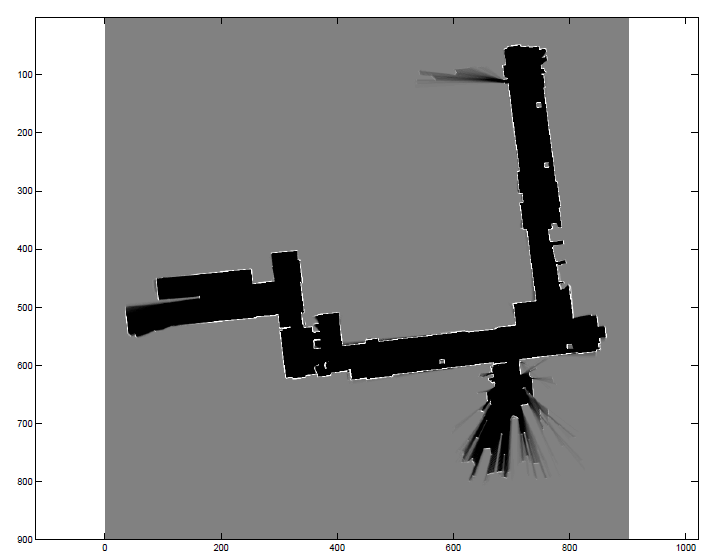## 1、地图的分类## 2、占据栅格地图构建算法

### 2.2、什么是占据栅格地图构建算法？### 2.3、举个例子验证占据栅格地图构建算法## 3、如何通过激光数据构建栅格地图？

### 3.1、算法核心依据### 3.3、算法步骤

//获取每一帧的激光雷达、机器人位姿数据
GeneralLaserScan scan = scans[i];
Eigen::Vector3d robotPose = robot_poses[i];

（2）计算该帧机器人位置的栅格序号

//获取该帧机器人位姿的栅格序号
GridIndex robotIndex = ConvertWorld2GridIndex(robotPose(0),robotPose(1));

//明确这里的世界坐标系world_x，不是真实的世界坐标系，而是像素坐标系，y轴与真实的世界坐标系相反，这样是laser_y加负号的原因
double laser_x =   dist * cos(theta + angle);
double laser_y =  -dist * sin(theta + angle);

//得到该激光扫描点，在世界坐标系下（像素坐标系下）的位置
double world_x = laser_x + robotPose(0);
double world_y = laser_y + robotPose(1);

//将该激光扫描点在世界坐标系下的位置，转化为栅格序号
GridIndex mapIndex = ConvertWorld2GridIndex(world_x,world_y);
 

//从机器人的栅格序号到该激光扫描点的栅格序号划线
//目的：找到两点之间途径的空闲栅格，将栅格序号存入std::vector<GridIndex>中
std::vector<GridIndex> freeIndex = TraceLine(robotIndex.x,robotIndex.y,mapIndex.x,mapIndex.y);data += mapParams.log_free;//log_free=-1，data将变小

data += mapParams.log_occ;//log_occ=2，data将变大

（4）结束

## 4、占据栅格地图构建算法c++实现

本算法功能包，给出了详细的中文注释，是提升c++编程技能的阅读良品

//占据栅格地图构建算法
//输入激光雷达数据和机器人位姿数据
//目的：通过遍历所有帧数据，为pMap[]中的每个穿过的空闲栅格或者击中栅格赋新值，中间有个计算方法，也就是占用栅格地图构建的理论实现
void OccupanyMapping(std::vector<GeneralLaserScan>& scans,std::vector<Eigen::Vector3d>& robot_poses)
{
std::cout <<"Scans Size:"<<scans.size()<<std::endl;
std::cout <<"Poses Size:"<<robot_poses.size()<<std::endl;

//遍历所有帧激光雷达数据
for(int i = 0; i < scans.size();i++)
{
//获取每一帧的激光雷达、机器人位姿数据
GeneralLaserScan scan = scans[i];
Eigen::Vector3d robotPose = robot_poses[i];

//获取该帧机器人位姿的栅格序号
GridIndex robotIndex = ConvertWorld2GridIndex(robotPose(0),robotPose(1));

//判断该帧机器人位姿的栅格序号，是否在自己设定的栅格地图范围内
if(isValidGridIndex(robotIndex) == false) continue;

//遍历该帧激光雷达数据所有扫描点
for(int id = 0; id < scan.range_readings.size();id++)
{
//取出该激光雷达扫描点的距离和角度
//剔除异常数据，跳过该次循环，不作处理
if(std::isinf(dist) || std::isnan(dist)) continue;
//机器人航向角，机器人x轴与世界坐标系x轴夹角
double theta = robotPose(2);

//在旋转过后（与世界坐标系（像素坐标系下）平行）的激光雷达坐标系下的坐标x,y
//该开始一直不理解这个为啥laser_y要加一个负号
//明确激光雷达数据的角度逆时针变化
//明确机器人航向角与世界坐标系x轴呈逆时针变化
//明确这里的世界坐标系world_x，不是真实的世界坐标系，而是像素坐标系，y轴与真实的世界坐标系相反，这样是laser_y加负号的原因
double laser_x =   dist * cos(theta + angle);
double laser_y =  -dist * sin(theta + angle);

//得到该激光扫描点，在世界坐标系下（像素坐标系下）的位置
double world_x = laser_x + robotPose(0);
double world_y = laser_y + robotPose(1);

//将该激光扫描点在世界坐标系下的位置，转化为栅格序号
GridIndex mapIndex = ConvertWorld2GridIndex(world_x,world_y);

//判断该激光扫描点的栅格序号，是否在自己设定的栅格地图900x900范围内，如果不在则跳过
if(isValidGridIndex(mapIndex) == false)continue;

//从机器人的栅格序号到该激光扫描点的栅格序号划线
//目的：找到两点之间途径的空闲栅格，将栅格序号存入std::vector<GridIndex>中
std::vector<GridIndex> freeIndex = TraceLine(robotIndex.x,robotIndex.y,mapIndex.x,mapIndex.y);

//遍历该扫描激光点通过的所有空闲栅格
for(int k = 0; k < freeIndex.size();k++)
{
GridIndex tmpIndex = freeIndex[k];
//将空闲栅格的栅格序号，转化到数组序号,该数组用于存储每一个栅格的数据
int linearIndex = GridIndexToLinearIndex(tmpIndex);
//取出该栅格代表的数据
int data = pMap[linearIndex];
//根据栅格空闲规则，执行data += mapParams.log_free;
if(data > 0)//默认data=50
data += mapParams.log_free;//log_free=-1，data将变小
else
data = 0;
//给该空闲栅格赋新值，最小为0
pMap[linearIndex] = data;
}
//更新该激光扫描点集中的栅格，
int tmpIndex = GridIndexToLinearIndex(mapIndex);
int data = pMap[tmpIndex];
//根据栅格击中规则，执行data += mapParams.log_occ;
if(data < 100)//默认data=50
data += mapParams.log_occ;//log_occ=2，data将变大
else
data = 100;
//给击中的栅格赋新值，最大100
pMap[tmpIndex] = data;
//到这里，对一个位姿下的一个激光扫描数据经过的空闲栅格和击中栅格的pMap进行了重新赋值
}
//到这里，对一个位姿下的一帧激光扫描数据经过的空闲栅格和击中栅格进行了重新赋值
}
//到这里，对所有帧激光扫描数据经过的空闲栅格和击中栅格进行了重新赋值
}

//从世界坐标系转换到栅格坐标系，主要是存在一个分辨率
//比如resolution = 0.04，世界坐标系下，单位1在栅格坐标系可以表示1/resolution=25个栅格
//目的：将机器人的实际位置，在900x900的栅格地图中找到对应的栅格序号，返回GridIndex对象
GridIndex ConvertWorld2GridIndex(double x,double y)
{
GridIndex index;
//ceil()向上取整函数
index.x = std::ceil((x - mapParams.origin_x) / mapParams.resolution) + mapParams.offset_x;
index.y = std::ceil((y - mapParams.origin_y) / mapParams.resolution) + mapParams.offset_y;

return index;
}
//2D画线算法　来进行计算两个点之间的grid cell
//目的：找到两点之间途径的空闲栅格，将栅格序号存入std::vector<GridIndex>中
std::vector<GridIndex> TraceLine(int x0, int y0, int x1, int y1)
{
GridIndex tmpIndex;
std::vector<GridIndex> gridIndexVector;

bool steep = abs(y1 - y0) > abs(x1 - x0);
if (steep)
{
std::swap(x0, y0);
std::swap(x1, y1);
}
if (x0 > x1)
{
std::swap(x0, x1);
std::swap(y0, y1);
}

int deltaX = x1 - x0;
int deltaY = abs(y1 - y0);
int error = 0;
int ystep;
int y = y0;

if (y0 < y1)
{
ystep = 1;
}
else
{
ystep = -1;
}

int pointX;
int pointY;
for (int x = x0; x <= x1; x++)
{
if (steep)
{
pointX = y;
pointY = x;
}
else
{
pointX = x;
pointY = y;
}

error += deltaY;

if (2 * error >= deltaX)
{
y += ystep;
error -= deltaX;
}

//不包含最后一个点．
if(pointX == x1 && pointY == y1) continue;

//保存所有的点
tmpIndex.SetIndex(pointX,pointY);

gridIndexVector.push_back(tmpIndex);
}

return gridIndexVector;
}

## 5、占据栅格地图构建算法matlab实现

本算法功能包，给出了详细的中文注释，是提升matlab编程技能的阅读良品。

function myMap = occGridMapping(ranges, scanAngles, pose, param)

resol = param.resol; % the number of grids for 1 meter.
myMap = zeros(param.size); % the initial map size in pixels
origin = param.origin; % the origin of the map in pixels

% Log-odd parameters
lo_occ = param.lo_occ;
lo_free = param.lo_free;
lo_max = param.lo_max;
lo_min = param.lo_min;

lidarn = size(scanAngles,1); % number of rays per timestamp
N = size(ranges,2); % number of timestamp

for i = 1:N % for each timestamp
theta = pose(3,i); % orientation of robot
% coordinate of robot in real world
x = pose(1,i);
y = pose(2,i);

% local coordinates of occupied points in real world
local_occs = [ranges(:,i).*cos(scanAngles+theta), -ranges(:,i).*sin(scanAngles+theta)];

% coordinate of robot in metric map
grid_rob = ceil(resol * [x; y]);

% calc coordinates of occupied and free points in metric map
for j=1:lidarn
real_occ = local_occs(j,:) + [x, y]; % global coordinate of occ in real world
grid_occ = ceil(resol * real_occ); % coordinate of occ in metric map

% coordinates of free in metric map (by breshnham's algorithm)
[freex, freey] = bresenham(grid_rob(1),grid_rob(2),grid_occ(1),grid_occ(2));
% convert coordinate to offset to array
free = sub2ind(size(myMap),freey+origin(2),freex+origin(1));
occ = sub2ind(size(myMap), grid_occ(2)+origin(2), grid_occ(1)+origin(1));
% update metric map
myMap(free) = myMap(free) - lo_free;
myMap(occ) = myMap(occ) + lo_occ;
end
end
% reassign value if out of range
myMap(myMap < lo_min) = lo_min;
myMap(myMap > lo_max) = lo_max;